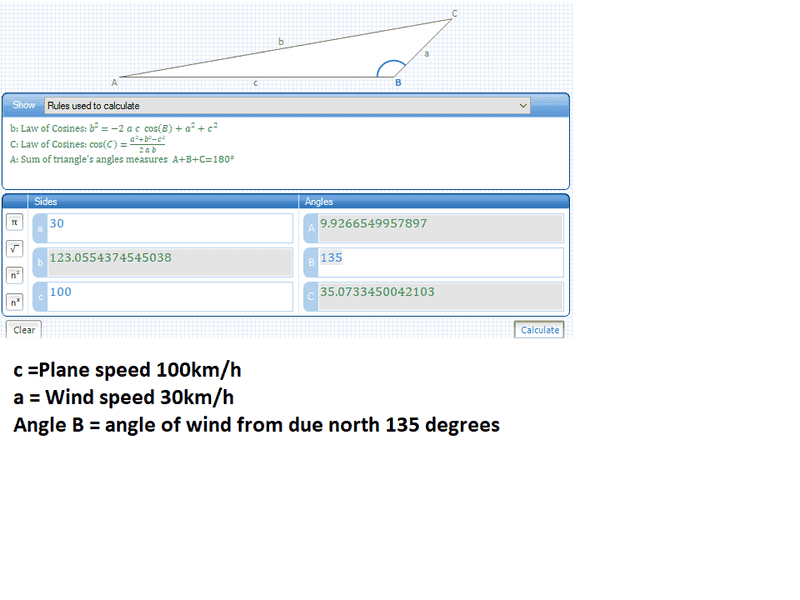# How do I do this trigonometry vector calculation?

• Jan Berkhout
In summary: The direction the plane must head to fly north is 9.93 degrees. Its speed relative to the ground is 123km/h.

## Homework Statement

A pilot wishes to fly at maximum speed due north. The plane can fly at 100km/h in still air. A 30km/h wind blows from the south-east.
Calculate:
a) The direction the plane must head to fly north.
b) Its speed relative to the ground.

## Homework Equations

Sine Rule: a/SinA=b/SinB=c/SinC
Cosine Rule: a2=b2+c2-2acCosA

## The Attempt at a Solution

I attached a photo because I didn't know how to do maths on here.
The answer I got was 9.93 degrees and 123km/hhttps://pasteboard.co/HwvgTjt.png
The answers from the sheet I'm working off say 12.24 degrees and 119km/h. And I don't understand how their way is correct. To get the angle they did: sin45/100=sinθ/30 If 100 is the planes speed it wouldn't be opposite to the angle of the direction of the wind.

#### Attachments

Last edited by a moderator:
Your triangle scheme is not correct. According to your scheme, the plane final speed (which is represented by the side b=(AC)) isn't towards north but somewhere between North-Northeast. Make again the triangle in such a way that the side b is vertical towards north and has magnitude unknown which we wish to find, side a is the direction of wind (from south east to northwest, makes 45 degrees angle with side b) and side c has magnitude 100 and direction unknow theta which we wish to find.

It is useful to memorise a vector equation that your relative motion triangles must always obey:

##v_b\ =\ v_a\ +\ v_{b\ rel\ a}##

and remember that to show this vector addition, the two vectors being summed need to be drawn head-to-tail.

You are making things difficult for yourself if you don't draw North as heading vertically up the page.

Jan Berkhout said:
I attached a photo because I didn't know how to do maths on here.
The answer I got was 9.93 degrees and 123km/h

In your diagram, you can't assume angle B is 135 degrees. Angle B is the angle between the pilot's unknown direction and the direction of the wind.

The given information let's us deduce the angle between due North and the direction of the wind. So we can deduce angle C.

•Delta2
Thank you all! I approached this question wrong and I just couldn't see a different way to look at until you showed me! This often happens to me with physics questions, Cheers!

•Delta2